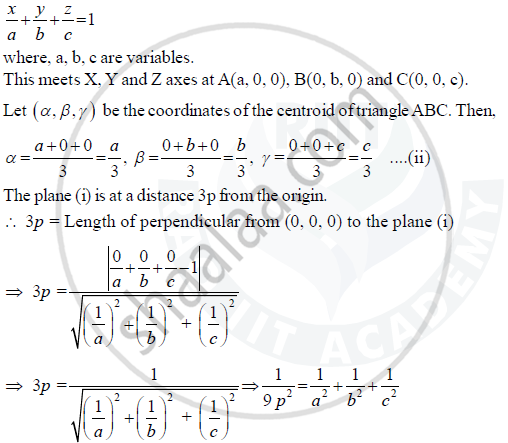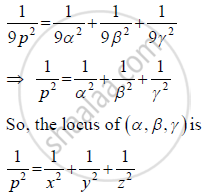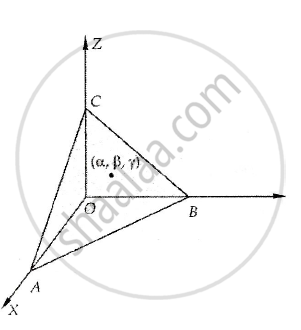Share

# A Variable Plane Which Remains at a Constant Distance 3p from the Origin Cuts the Coordinate Axes at A, B, C. Show that the Locus of the Centroid of Triangle Abc is 1/X^2 + 1/Y^2 + 1/Z^2 = 1/P^2 - CBSE (Commerce) Class 12 - Mathematics

ConceptPlane Intercept Form of the Equation of a Plane

#### Question

A variable plane which remains at a constant distance 3p from the origin cuts the coordinate axes at A, B, C. Show that the locus of the centroid of triangle ABC is 1/x^2 + 1/y^2 + 1/z^2 = 1/p^2

#### Solution

Let the equation of the plane beFrom (ii), we have

a = 3α ,b = 3β and c = 3γ

Substituting the values of a, b, c in (iii), we obtainIs there an error in this question or solution?

#### Video TutorialsVIEW ALL 

Solution A Variable Plane Which Remains at a Constant Distance 3p from the Origin Cuts the Coordinate Axes at A, B, C. Show that the Locus of the Centroid of Triangle Abc is 1/X^2 + 1/Y^2 + 1/Z^2 = 1/P^2 Concept: Plane - Intercept Form of the Equation of a Plane.
S